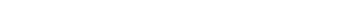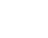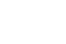# Kenya Certificate Of Secondary Education (KCSE 2008) Physics Paper 2

SECTION A (25 marks)
Answer ALL the questions in this section in the spaces provided.

1.  Figure 1 shows three points sources of light with an opaque object placed between them and the screen.Explain the nature of the shadow formed along B and C. (2 marks)

2. A leaf electroscope A is charged and placed on the bench. Another uncharged leaf electroscope B is placed on the same bench and moved close to A until the caps touch. State and explain what is observed on the leaves of A and B. (2 marks)

3. You are provided with the following.
A cell and a cell holder, as switch, a rheostat, an ammeter, a voltmeter and connecting wires. Draw a diagram for a circuit that could be used to investigate the variation of the potential difference across the cell with the current drawn from the cell. (1 mark)

4. An unmagnetized steel rod is clamped facing North-South direction and then hammered repeatedly for some time. When tested, it is found to be magnetized. Explain this observation. (2 marks)

5. The diagram in figure 2 shows an object placed infront of a converging lens. F and F' and the principal foci for the lens.The object is now moved along the principal axis until a virtual image is produced.

On the same diagram:

1. draw the object O in the new position along the principal axis;

2. sketch rays to show formation of the virtual image. (1 mark)

6. Figure 3 shows a flat screen made of iron clamped horizontally on the bench over the solenoid.When the switch is closed, the spring vibrates. Explain this observation. (3 marks)

7. Figure 4 shows a hack-saw blade clamped horizontally on a bench and the free end is made to vibrate about the rest position.The movementtakes 0.7 seconds.

Determine the frequency of vibration of the blade. (2 marks)

8.

Figure 5 shows wavefronts approaching the boundary between two media.The speed of the waves in medium (2) is higher than that in medium (1).

On the same diagram, complete the figure to show the wave fronts after crossing the boundary. (2 marks)

9.  Figure 6 shows a circuit in which a battery of negligible internal resistance, two resistors, a capacitor a voltmeter and a switch are connected.Giving a reason for your answer in each case, state the reading of the voltmeter, V, when the switch is
1. Open
2. Closed

10. A heating coil is rated 100W, 240V. At what rate would it dissipate energy if it is connected to a 220V supply? (3 marks)

11. Figure 7 shows how rays from a distant and near object are focused inside the human eye with a certain defect.Name the defect and state the cause of this defect. ( 1 mark)

12.

A narrow beam of electrons in a cathode ray oscilloscope (CRO) strike the screen producing a spot. State what is observed on the  if a low frequency a.c source is connected across the y-input of the CRO. (1 mark)

13. The accelerating potential of a certain X-ray tube is increased. State the change observed on the X-rays produced. (1 mark)

14. A radioactive isotope of copper decays to form an isotope of zinc as shown below. (2 marks)

SECTION B (55 marks)

Answer ALL the questions in this section in the spaces provided.

1. State one factor that affects the speed of sound in a solid. (1 mark)
2. An observer stands half-way between two vertical cliffs that re L metres apart. He moves directly towards once cliff and after a distance x = 10m from the centre, he strikes a gong, and measures the time interval, t, between the echoes heard from the two cliffs. He moves a further 10m and again strikes the gong and measures the time interval between the echoes. The process is repeated several times. The graph in Figure 8 shows the relation between the time interval, t, and the distance ,x , from the centre.1. From the graph, determine the value of x for which the interval was 0.55 s. (1 mark)
2. Given thatwhere v is the speed is of sound in air. Determine the value of v from the graph. (3 marks)
3. If the maximum time measured by the observer was t = 4.7s, determine the distance L between the cliffs. (3 marks)

3. A search boat uses a signal frequency 6.0× 104Hz to detect a sunken ship directly below. Two reflected signals are received; one after 0.1 seconds from the sunken boat and the other after 0.14 seconds from the sea bed. If the sea bed is 98m below the boat, determine:-
1. the speed of the signal in water. (3 marks)
You may use the value of v from (ii) above.

2. the depth of the sunken ship below the boat. (2 marks)

1. State two conditions necessary for the total internal reflection to occur. (2 marks)
2. Figure 9 shows a ray of light incident on the boundary between two media 1 and 2 at the angle of θ.Show that the refractive index from a ray of light travelling from medium 1 to 2 is given by:(2 marks)

3. Figure 10 shows a ray of light incident on one face of a block of ice of refractive index 1.31 and totally reflected at the adjacent face.Determine:
1. Angle Φ (2 marks)
2. angle x (1 mark)
3. angle θ the greatest angle for which the total internal refection is possible. (2 marks)

1. Three resisitors of resistance 2.0Ω, 4.0Ω and 6.0Ω are connected together in a circuit. Draw a circuit diagram to show the arrangements of the resistors which gives:
1. effective resistance of 3.0Ω (1 mark)
2. minimum resistance. (1 mark)

2. In figure 11 the voltmeter reads 2.1V when the switch is open. When the switch is closed the voltmeter reads 1.8V and the ammeter reads 0.1A.Determine:
1. the e.m.f of the cell: (1 mark)
2. the internal resistance of the cell. (3 marks)
3. the resistance of the lamp. (2 marks)

1. Figure 12 shows two circuits close to each other.When the switch is closed, the galvanometer shows a reading and then returns to zero. When the switch is open, the galvanometer shows a reading in the opposite direction and then returns to zero. Explain these observations. (3 marks)

2. Explain how energy losses in a transformer are reduced by:
1. a soft-iron core; (2 marks)
2. a laminated core. (2 marks)

3. An ideal transformer has 2000 turns in the primary circuit and 200 turns in the secondary circuit. When the primary circuit is connected to a 400V a.c. source, the power delivered to a resistor in the secondary circuit is found to be 800W.
1. the secondary circuit;
2. the primary circuit.                                        (6 marks)

1. X- rays are used for detecting cracks inside metal beams.
1. State the type of X-rays used. (1 mark)

2.

Give a reason for your reason in (i) above. (1 mark)

2. Figure 13 shows the features of an X-ray tube.1. Name the parts labelled A and B. (2 marks)
2. Explain how change in the potential across PQ changes the intensity of the X-rays produced in the tube. (2 marks)
3. During the operation of the tube, the target becomes very hot. Explain how the heat is caused. (1 mark)
4. What property of lead makes it suitable for use as shielding material? (1 mark)

3. In a certain X-ray tube, the electrons are accelerated by the pd of 12000V. Assuming all the energy goes to produce X-rays, determine the frequency of the X-rays produced. (Plank's constant h= 6.62× 10-34 Js and charge on an electron, e= 1.6×10-19 C). (4 marks)

#### Download Kenya Certificate Of Secondary Education (KCSE 2008) Physics Paper 2.

• ✔ To read offline at any time.
• ✔ To Print at your convenience
• ✔ Share Easily with Friends / Students